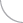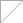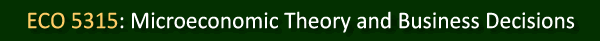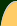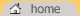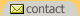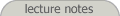Lecture notes for ECO 5315 will be posted in PowerPoint (either .ppt or .pptx) and/or Adobe Acrobat (.pdf) file formats. Spreadsheets which accompany these lectures will be posted in either Excel 2003 (.xls) or Excel 2007 (.xlsx) file formats. Mathematica player notebooks (.nbp) require the Mathematica Player. Chapter 1: Introduction to Managerial Economics (PowerPoint format, Acrobat Acrobat format) (October 1) Spreadsheets Chapter 2: Demand Theory and Chapter 4: Production Theory (PowerPoint format, Acrobat Acrobat format) (October 8) Spreadsheets Maximize Total Revenue (Chapter 2) Elasticity and Marginal Revenue (Chapter 2) Total, Average and Marginal Product (Chapter 4) Chapter 4: Production Theory (PowerPoint format, Acrobat Acrobat format) (October 15) Mathematica Player Notebook (Chapter 4): Production Function Example (2D and 3D) (Right Mouse Click, then "Save As"). Spreadsheets (Chapter 4) Chapter 5: The Analysis of Costs and Chapter 6: Perfect Competition (PowerPoint format, Acrobat Acrobat format) (October 22) Spreadsheets (Chapter 5) Chapter 7: Monopoly and Monopolistic Competition and Chapter 10: Oligopoly (PowerPoint format, Acrobat Acrobat format) (October 29) Midterm Review Session (November 5) Chapter 11: Game Theory (PowerPoint format, Acrobat Acrobat format) (November 16) Chapter 13: Risk Analysis (PowerPoint format, Acrobat Acrobat format) (November 23) Chapter 14: Principal-Agent Issues and Managerial Compensation and Chapter 15: Adverse Selection (November 30) (PowerPoint format, Acrobat Acrobat format) Spreadsheets (Chapter 14) Catch-up and Review (December 7)